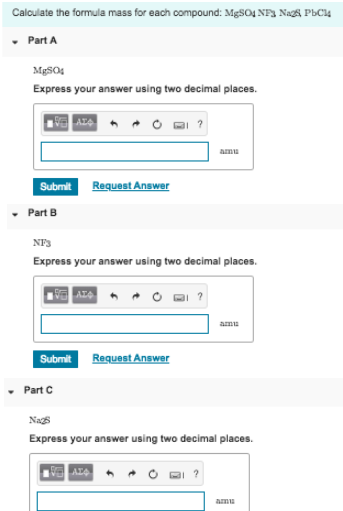# Problem: Calculate the formula mass for each compound: MgSO4, NF3, Na2S, Part A MgSO4 Express your answer using two decimal places. Part B NF3 Express your answer using two decimal places. Part C Na2S Express your answer using two decimal places

###### FREE Expert Solution
84% (403 ratings)###### Problem Details

Calculate the formula mass for each compound: MgSO4, NF3, Na2S,

Part A

MgSO4

Part B

NF3

Part C

Na2